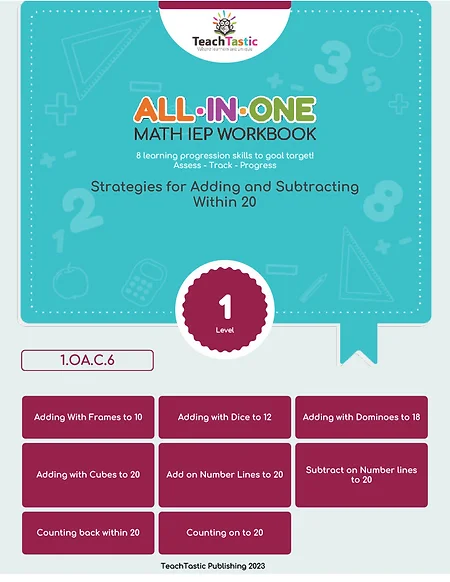top of page

# Lesson Plan

### First (1) - Math### LEARNING TARGET

• Students will be able to use 9's domino visuals to add sums up to 18.
• Students will be able to use the commutative property strategy to add two numbers.### LEARNING PROGRESSION

###### PREREQUISITE SKILL
1. Students will be able to identify numbers 1-6 on a die.
2. Students will be able to add two numbers together using the commutative property.
3. Students will be able to add sums up to 12 using dice visuals and the commutative property.
###### EXTENSION SKILL
• Students will be able to use connecting cube visuals to add numbers to 20.
• Students will be able to apply the commutative property strategy to add numbers to 20.
• Students will be able to solve addition problems involving numbers up to 20.
###### DURATION
• 45-55 minutes
###### MATERIALS
• 9's domino visuals (enough for each student)
• Whiteboard and markers
• Number cards (0-9)
• Worksheet
• Homework sheet (optional)
###### VOCABULARY
• Dominoes
• Sum
• Commutative property### TEACHING RESOURCESNo Centers or Task Cards Available

###### IEP GOAL WORKBOOKSIntroducing the Strategies for Adding and Subtracting Within 20 workbook, designed for special education teachers who want innovative and practical ways to teach and scaffold learning for students with IEP goals. With a focus on individualized support, this workbook provides a clear and concise approach to mastering addition and subtraction within 20.

###### WORKSHEET PACKTeach addition using commutative property and 9's domino visuals with our worksheet pack. Includes lesson plan for student progress monitoring in math.

###### 5 AND 1 INTERVENTIONSNo Interventions Available

###### GAMES###### ACTIVITIESNo Activities Available### LESSON INSTRUCTION

###### INTRODUCTION
1. Review with students what addition means and how to use the plus sign (+) to represent it.
2. Introduce the concept of using 9's domino visuals to add numbers.
3. Show students the 9's domino visuals and explain how they work.
4. Model an example of adding two numbers using the domino visuals.
###### INSTRUCTION
1. Demonstrate to students how to use the commutative property strategy to add two numbers.
2. Write an example problem on the board and show how to use the commutative property to solve it.
3. Practice this strategy with a few more problems.
###### GUIDED PRACTICE
1. Distribute the 9's domino visuals and number cards to each student.
2. Write a few addition problems on the board for students to solve using the domino visuals and the commutative property strategy.
3. Work through the problems together as a class.

###### INDEPENDENT PRACTICE
1. Distribute the worksheet to each student to complete independently.
2. Encourage students to use the domino visuals and the commutative property strategy to solve the problems.
3. Circulate around the room to provide support and guidance as needed.

###### HOMEWORK
1. Distribute the optional homework sheet to each student.
2. Instruct students to find objects around their home and use the domino visuals and commutative property strategy to add them together.
3. Remind students to bring their homework back to class to share with their classmates.

###### EXIT TICKET
1. Provide each student with a whiteboard and marker.
2. Instruct students to write an addition problem and solve it using the domino visuals and commutative property strategy.

###### ASSESSMENT
1. Ask students to share one addition problem they solved using the domino visuals and commutative property strategy.
2. Use their responses to assess their understanding of the lesson objectives.

###### CLOSURE
1. Review with students what they learned about adding sums to 18 using 9's domino visuals and the commutative property strategy.
2. Encourage students to practice using these strategies to add numbers at home.

###### EXTENSION
1. Students can practice adding larger numbers using the domino visuals and commutative property strategy.
2. Students can practice subtracting numbers using the same visuals and strategy.
###### INTERVENTION
1. For students who struggle with fine motor skills, larger and easier to grasp domino visuals can be used.
2. For students who need extra support, a visual aid or diagram can be provided to help them understand the concept of adding numbers using the domino
3. visuals and commutative property strategy.
4. For students who need additional practice, they can work on more problems with smaller numbers before moving on to larger ones.
###### VIDEOS

No Video Available### TEACHING TIPS

• Use hands-on activities and manipulatives to make math more engaging and accessible for young learners.
• Model problem-solving strategies and encourage students to ask questions and share their thinking.
• Scaffold instruction and provide differentiated support to help students who may be struggling with the material.### STUDENT MISCONCEPTIONS

• Confusing the plus and minus signs, leading to errors in addition and subtraction.
• Struggling to understand the concept of regrouping, particularly when adding larger numbers.
• Difficulty with memorizing basic addition facts, which can impact their ability to solve problems quickly and efficiently.### STANDARD

##### Common Core Standard:

1.OA.C.6 - Add and subtract within 20, demonstrating fluency for addition and subtraction within 10. Use strategies such as counting on; making ten; decomposing a number leading to a ten; using the relationship between addition and subtraction.

bottom of page